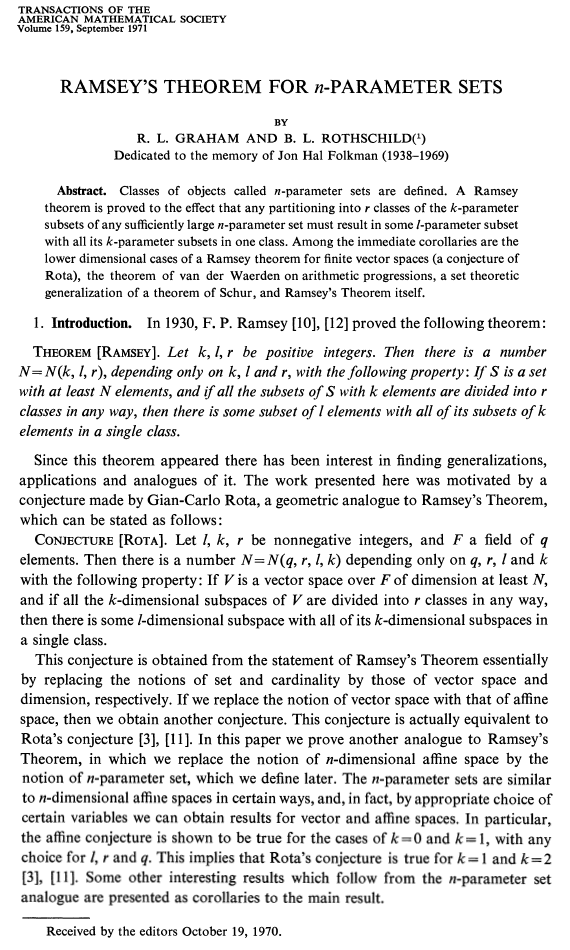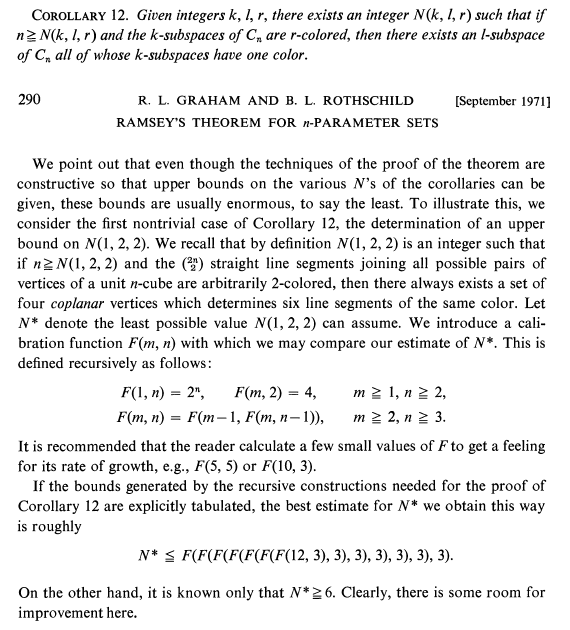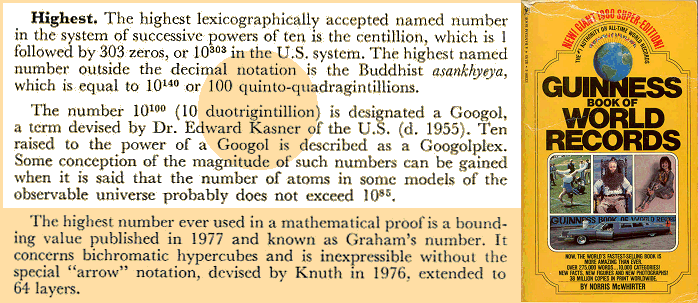## Gardner on Graham's number## Graham on Graham's numberF(2,n) = F(1,F(2,n-1)) = 2^F(2,n-1)
== 2^..4 :n-2 = 2^^n
F(3,n) = F(2,F(3,n-1)) = 2^^F(3,n-1)
== 2^^..4 :n-2 = 2^^^n

F(m,n) = 2^..n ^:m = 2→n→m

a→b→c+1→2 = a→b→(a→b→c→2)
== a→b→(..a→b→1→2.) :c:
= a→b→(..a^b.) :c:

N* ≤ 2→3→(..12.) :7:
< 4→2→8→2 == 4→2→(..16.) :7:

## Guinness on Graham's number## Summary

Graham's number record explained by Gardner
GN' ≤ 3→3→(..4.) :64:
< 2→3→65→2 = 2→3→(..8.) :64:

Graham & Rothschild's original estimate was less
GN* ≤ 2→3→(..12.) :7:
< 4→2→8→2  <  2→3→9→2

New bounds for Graham's number of hypercube dimensions
13 ≤ GN ≤ 2→6→3        [as of September 2014]